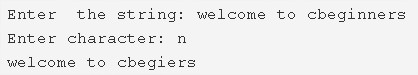# C Program To Remove All Occurrences Of A Character From String | C Programs

C program to remove all occurrences of a character with another in a string – In this article, we will examine the various means to remove all occurrences of a character with another in a string in C programming.

The ways explained in this piece are as follows:

• Using Standard Model
• Using Function
• Using Recursion

A string is nothing but an array of characters. The value of a string is determined by the terminating character. Its value is considered to be 0.As we can see in the image uploaded above, a string is to be entered first up.

The string entered here is “welcome to cbeginners”.

We need to stipulate which character needs to be removed.

The character ‘n’ is chosen to be removed here from the whole string.

So, after removing, the string became:

“welcome to cbegiers”

Thus, the techniques used to do so in C programming are as follows:

## Using Standard Method

1. Initialize temp=1,k=0.

2) Read the user entered string using gets(s). read the character which we want to remove

3) Read the character which we want to remove it’s all occurrences from the string, using getchar() function.

4) The for loop iterates through the string with the structure for(i=0;s[i];i++) until the last character of the string becomes to null.

a) Initialize s[i]=s[i+k]

b) If s[i] match with the entered character then increase the k value and decrease the i value.

We are shifting the character which is right to the removed character, to one position left.

4) Print the string which contains the elements without the removed character and it’s all occurrences.

Output:

### Using Function

1. The main() calls the deletechar(char *s, char c) function by passing the string, character as arguments to the function.

2) The function deletechar(char *s, char c) will remove all occurrences of the entered character from the string.

a) for loop iterates through the string with the structure for(i=0;s[i];i++)

b) If finds each occurrence of the entered character and at each occurrence, i decreased by 1 and k increased by 1 and it shifts the element right to the removed element to one position left as s[i]=s[i+k].

3) Then main() function prints the string which contains the elements except, all occurrences of the removed element.

Output:

#### Using Recursion

1. The main() calls the deletechar(char *s,char c) function which is recursive function.

2) The function deletechar(char *s,char c)Initialize the variables i=0 and k=0.

Initialize the variables i=0 and k=0.

a) If the element at s[i] is null then it returns back to the main function.

b) If the element exists then

b.1) initialize s[i]=s[i+k].

b.2) If the entered character is equal to s[i] then increase the k value and decrease the i value.

b.3) Increase the i value and call the function itself to find the next occurrence of the entered character and removes its occurrence from the given string.

The function calls itself recursively until the last character of the string.

3) Print the string after removing all occurrences of the entered character.

Output: